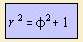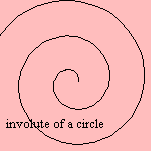# involute of a circle

## spiralThe curve is the involute of a circle 1).
Some authors add the points (-y, x) to the curve as shown on the page.
Sometimes the curve is called the evolute.

For large angles (>>1), the curve approximates the Archimedes' spiral, this curve also being the pedal of the involute of a circle.
When the curve is rolling over a line, then the path of the center is a parabola. And its pedal is an Archimedes' spiral.

The curvature of the curve is equal to the reciprocal root of the arc length s: dφ/ds = 1/√s.
Therefore the Cesaró equation - which gives the relation between the radius of curvature R and the arc length s - has the elegant form R2 = s.

When the path of the curve is followed in a linear way (in time), the rotation speed is constant. While this is the reverse definition as for the clothoid, the involute of a circle is also called the anti-clothoid.

Huygens used the curve in his experiments to have a cycloid swing in his pendulum clocks.
In mechanical engineering the curve is used for the profile of a gear-wheel, in the situation of non-parallel axes.

notes

1) In French: développante du cercle; in German: Kreisevolvente; in Dutch: cirkelevolvente.